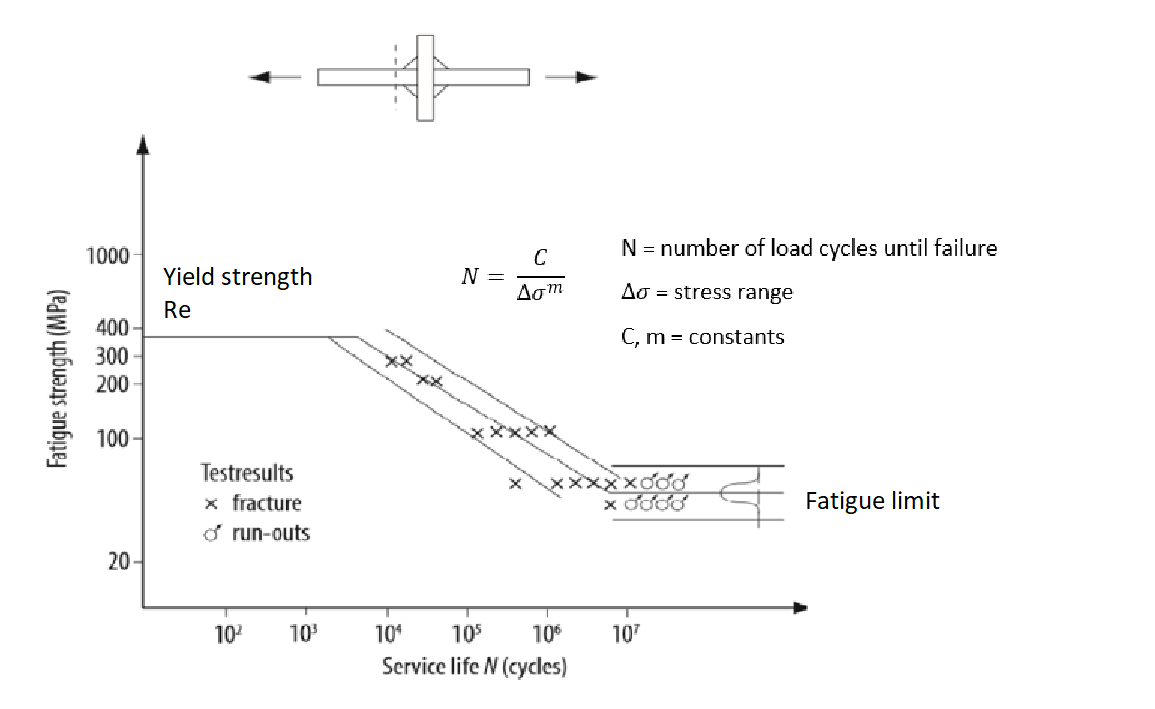Fatigue testing normally results in a scatter of values that seemingly has a huge span in range. However, if they are plotted in an SN-diagram the data can be analysed. The SN-curve plots the fatigue strength and the service life of the specimens according to picture 1.

Fatigue strength decreases as the number of load cycles increases. In a log-log diagram there is a linear equation that describes how the fatigue strength depends on the number of cycles.

For a very low number of cycles the static strength is limiting the strength level. The yield strength is then often the design strength, i.e. you have a static load case. Note that the fatigue strength can be much lower than the yield strength also for a low number of cycles, especially when you have stress raisers as welds in the design.

Under some special conditions there sometimes also exists what you call a fatigue limit, i.e. a value of the fatigue strength that is constant over a certain number of cycles.

In practice, often a structure is subject to a fatigue load if the number of cycles are over 1000 cycles.

The FAT-value is simply the characteristic fatigue strength of a test specimen at 2.000.000 cycles and full load spectrum (constant stress range all the life time).

The slope of the fatigue curve (the value m of the SN-curve) is different depending on the specimen. If it is pure base material with no defects the slope is often 5, if it is a welded specimen the slope is often 3.Picture 1: Fatigue strength versus number of load cycles, the SN-curve

The fatigue strength of the design at a certain critical point is described by a straight line in a SN-diagram. The slope of the SN-curve is m=3 for welded joints and m=5 for base material. The FAT-value at 2 million cycles and constant amplitude can be as low as 35 MPa for a fillet weld joint, with an unfavourable geometry. If the load spectrum is very thin and high strength base material can be utilised in the critical points, the fatigue strength can be very high and the static design criteria using the yield strength might determine the load capacity. A varying slope of the SN-curve with increasing FAT-values (earlier called joint class C-values) has been discussed, picture 2.Picture 2: Curves with adjusted slope with regards to increased joint class. However, the slope of SN-curve is in FATcomp m=3 for welded joints and m=5 for base material (according to European standard 2017).